# GSEB Solutions Class 6 Maths Chapter 4 Basic Geometrical Ideas Ex 4.1

Gujarat Board GSEB Textbook Solutions Class 6 Maths Chapter 4 Basic Geometrical Ideas Ex 4.1 Textbook Questions and Answers.

## Gujarat Board Textbook Solutions Class 6 Maths Chapter 4 Basic Geometrical Ideas Ex 4.1

Question 1.
Use the figure to name.
(a) Five points
(b) A line
(c) Four rays
(d) Five line segments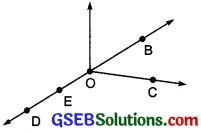Solution:
(a) Five points are: B, C, D, E and O
(b) A line is: $$\stackrel{\leftrightarrow}{DB}$$ (or $$\stackrel{\leftrightarrow}{BD}$$)
(c) Four rays are: $$\overrightarrow{\mathrm{OB}}$$, $$\overrightarrow{\mathrm{OC}}$$, $$\overrightarrow{\mathrm{OD}}$$ and $$\overrightarrow{\mathrm{OE}}$$
(d) Five line segments are: $$\overline{\mathrm{OB}}$$, $$\overline{\mathrm{OC}}$$, $$\overline{\mathrm{OD}}$$, $$\overline{\mathrm{DE}}$$ and $$\overline{\mathrm{OE}}$$.Question 2.
Name the line given in all possible A B C D (twelve) ways, choosing only two letters at a time from the four given.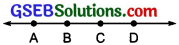Solution:
The given line can be named in the following ways: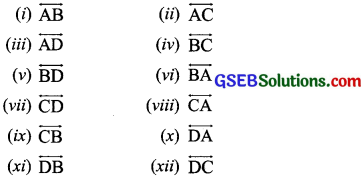Question 3.
Use the figure to name:
(a) Line containing point E.
(b) Line passing through A.
(c) Line on which O lies.
(d) Two pairs of intersecting lines.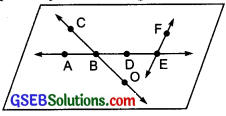Solution: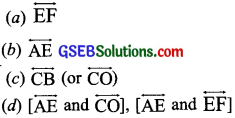Question 4.
How many lines can pass through
(a) one given point?
(b) two given points?
Solution:
(a) An infinite number of lines and
(b) Only one lineQuestion 5.
Draw a rough figure and label suitably in each of the following cases :
(a) Point P lies on $$\overline{\mathrm{AB}}$$.
(b) $$\stackrel{\leftrightarrow}{XY}$$ and $$\stackrel{\leftrightarrow}{PQ}$$ intersect at M.
(c) Line l contains E and F but not D.
(d) $$\stackrel{\leftrightarrow}{OP}$$ and $$\stackrel{\leftrightarrow}{OQ}$$ meet at O.
Solution: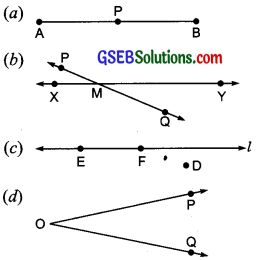Question 6.
Consider the following figure of line . Say whether following statements are true or false in context of the given figure.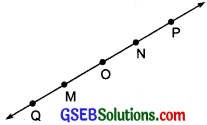(a) Q, M, O, N, P are points on the line $$\stackrel{\leftrightarrow}{MN}$$.
(b) M, O, N are points on a line segment $$\overline{\mathrm{MN}}$$.
(c) M and N are endpoints of line segment $$\overline{\mathrm{MN}}$$.
(d) O and N are end points of line segment $$\overline{\mathrm{OP}}$$.
(e) M is one of the end points of line segment $$\overline{\mathrm{QO}}$$.
(f) M is point on ray $$\stackrel{\leftrightarrow}{QP}$$.
(g) Ray $$\stackrel{\leftrightarrow}{QP}$$ is dijferent from ray $$\stackrel{\leftrightarrow}{QP}$$.
(h) Ray $$\stackrel{\leftrightarrow}{QP}$$ is same as ray $$\stackrel{\leftrightarrow}{QP}$$
(i) Ray is not opposite to ray $$\stackrel{\leftrightarrow}{QP}$$
(j) O is not an initial point of $$\stackrel{\leftrightarrow}{QP}$$.
(k) N is the initial point of $$\stackrel{\leftrightarrow}{NP}$$ and $$\stackrel{\leftrightarrow}{NM}$$.
Solution:
(a) True
(b) True
(c) True
(d) False
(e) False
(f) False
(g) True
(h) False
(i) False
(j) False
(k) True